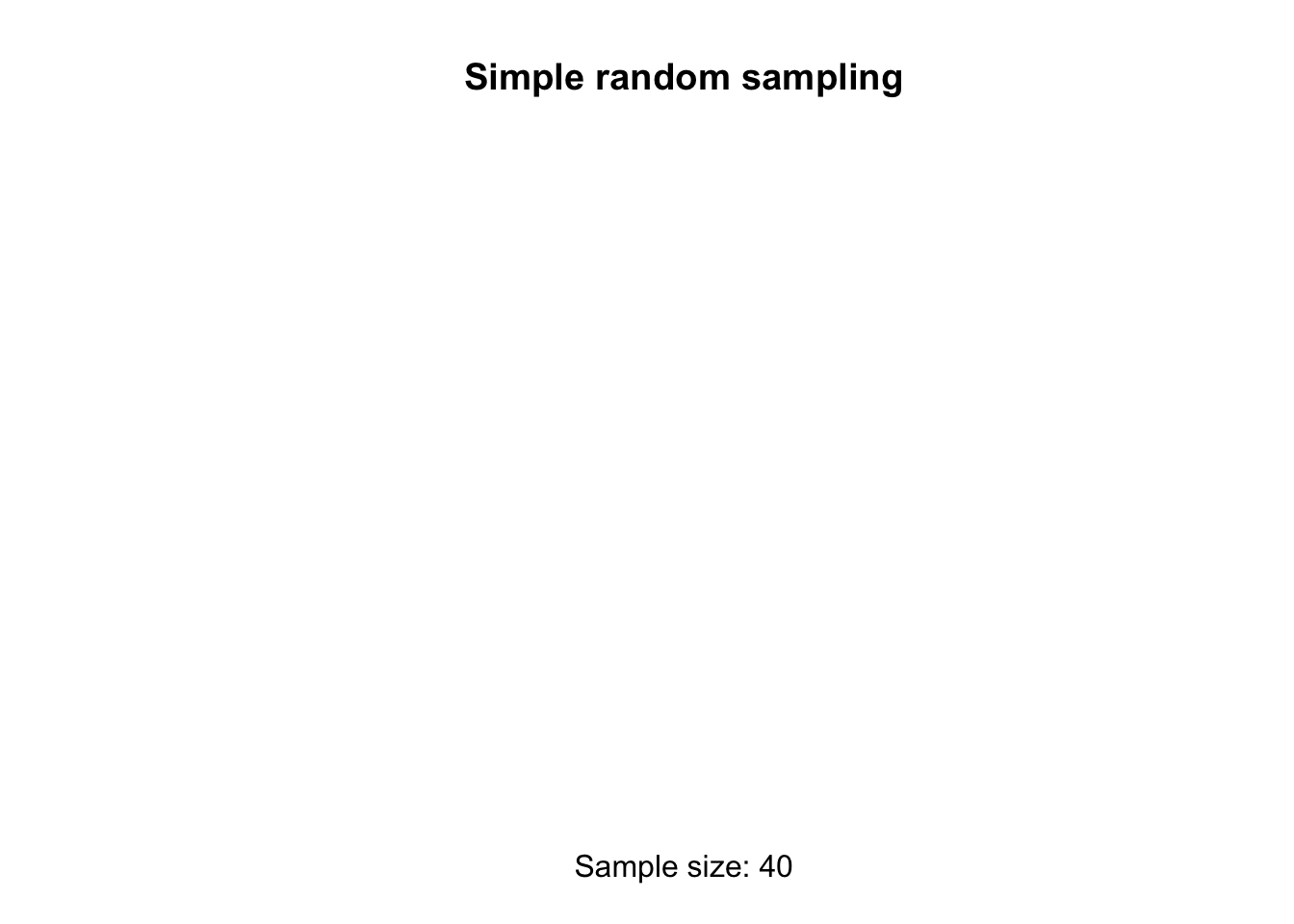## 5.4 Simple random samplingDefinition 5.4 In a simple random sample, every possible sample of the same size has same chance of being selected.

A simple random sample is chosen from a list of all members of the population (the sampling frame) using tables of random numbers (Appendix B.1) or websites like https://www.random.org. A smaller version of this webpage, which only generates one number at a time, is shown below; just press Generate. The numbers generated by this widget come from the true random number generator at RANDOM.ORG. (The webpage generates as many numbers as you want all at the same time.)

Definition 5.5 (Sampling frame) The sampling frame is a list of all the members of the population (the individuals, or cases, or subjects).

Often, establishing the sampling frame is difficult or impossible, and so finding a random sample is also difficult.

For example, to study a simple random sample of wombats would require having a list of all wombats, so some could be selected using random number tables. This is clearly absurd, and other random sampling methods, such as special ecological sampling methods, would be used instead .

Other good (random) sampling methods use a system to select randomly, rather than by human choice (discussed in the following sections).

This book always assumes simple random samples, for simplicity, unless otherwise noted.Example 5.4 (Simple random sampling) Suppose we are interested in this RQ:

For students at a large course at a particular university, is the average number of letters typed on a keyboard in 10 seconds the same for females and males?

Suppose a sample of 40 students is needed. The sampling frame is the list of all students enrolled. Obtaining the sampling frame is feasible here (lecturers have access to this information for grading).

Think 5.2 (Simple random sampling) Suppose budget and time constraints mean only 40 students can be selected for the study above.

Describe how to use the course enrolment list to find a simple random sample of 40 students to study.### References

Manly BFJ, Alberto JAN. Introduction to ecological sampling. CRC Press; 2014.
Yang P, Chan M, Carver S, Hu D. How do wombats make cubed poo? Bulletin of the American Physical Society. APS; 2018;